# Chemical Reaction Effects on MHD Slip Flow of Convective Nanofluid over a Vertical Plate Embedded in Porous Medium with Heat Source/Sink

Chemical Reaction Effects on MHD Slip Flow of Convective Nanofluid over a Vertical Plate Embedded in Porous Medium with Heat Source/Sink
Department of Mathematics, Gandhi Institute for Technology, Bhubaneswar 752054, India

Department of Mathematics, Siksha’O’ Anusandhan (Deemed to be University), Bhubaneswar 751030, India

Corresponding Author Email:
kharabela1983@gmail.com
Page:
43-50
|
DOI:
https://doi.org/10.18280/mmc_b.882-401
5 April 2019
|
Accepted:
6 July 2019
|
Published:
30 December 2019
| Citation

OPEN ACCESS

Abstract:

In this present flow model we study the MHD flow, heat and mass transfer of an electrically conducting nanofluid past a semi-infinite vertical flat plate subject to convective boundary condition, energy dissipation, heat source/sink and chemical reaction. Using similarity transformations, we derive a set of non-linear ordinary differential equations governing the flow which are solved numerically by fourth order Runge-Kutta method with shooting technique. The velocity, temperature, concentration and nanoparticle volume fraction profiles are affected by a number of thermo-physical parameters are presented graphically. The shearing stress at the plate, rate of heat and mass transfer are also studied. The outcome of the present analysis is to regulate the Biot number to control the cooling or heating mechanism at the plate i.e. to increase/decrease the rate of heat transfer.

Keywords:

MHD, nanofluid, thermal radiation, viscous dissipation, chemical reaction

1. Introduction

A nanofluid is a blend of base fluid and nanometer sized particles uniformly suspended in the fluid. Typically conductive fluids such as water, ethylene glycol and mineral salts are used as base fluid. The nanoparticles are made of metals (Al, Cu), carbides (SiC), oxides (Al2O3), nitrides (AlN, SiN), or nonmetals (carbon nanotubes, graphite).  The nanofluids are of enormous use in many manufacturing and engineering applications such as melt polymers, electronics cooling, solar collectors, biological solutions, food mixing, nuclear applications. Nanofluid first coined by Choi . Choi et al.  noticed that the effect of a small amount of nanoparticles added to any ordinary fluid (less than one percent by volume) enhances approximately twice the thermal conductivity of the base fluid. Buongiorno  derived a nanofluid model in which Brownian diffusion and thermophoresis slip mechanism are used to augment thermal property of the base fluid. Researches reveal that the slip flow exists for small characteristic size of the flow system or flow with very low pressure. The part of the slip may occur on a stationary and moving boundary when the fluid is particulate such as emulsions and suspensions. Navier  comprised a boundary condition which states that the component of tangential fluid velocity to the boundary walls is directly proportional to tangential stress. An analytical solution on the natural convective boundary layer nanofluid flow past a vertical plate was described by Kuznetsov and Nield . They found that Brownian motion of nanoparticles plays a key role on nanoscale mechanism augmenting the heat transfer properties.

The MHD flow on boundary layer over a stretching surface has wide applications in chemical, mechanical, industrial and manufacturing processes such as aero dynamics, polymer production, metal casting etc. Hussain et al.  have studied the thermal radiation effect on magneto-nanofluid over an exponentially stretching sheet. Nayak  studied the MHD flow and heat transfer on stretched vertical permeable surface considering thermal radiation and chemical reaction. Swain et al.  have studied the MHD heat and mass transfer on stretching sheet with variable viscosity as well as thermal conductivity. The thermo-diffusion has also been taken care of. The diffusion of mass because of temperature gradient is called Soret or thermo-diffusion effects and this effect is considerable for large density differences between the fluid layers of diffusing species and surrounding fluid medium. The Soret and Dufour effect on mixed convection heat and mass transfer are studied by Pal and Chatterjee . Makinde and Aziz  studied numerically boundary layer flow of a nanofluid past a stretching sheet subject to a convective thermal boundary condition. Further, RamReddy et al.  studied the effects of mixed convection flow taking a vertical plate subject to a convective boundary condition. They solved the modeled equations numerically by applying implicit iterative finite difference method. Nayak et al.  investigated the Soret and Dufour effects in the hydro-magnetic unsteady flow by a mixed convection past a stretching sheet in a porous medium in the presence of chemical reaction. Swain et al.  have studied the impact of heat source and chemical reaction on MHD flow of viscoelastic nanofluid over a stretching sheet in a porous medium. Rama and Goyal  investigated non-Newtonian nanofluid flow over a permeable sheet with heat generation and velocity slip in presence of magnetic field. Barik et al.  analyzed the effect of chemical reaction and heat source on MHD flow of visco-elastic fluid past an exponentially accelerated vertical plate embedded in a porous medium. Nayak et al.  examined the flow and mass transfer analysis of a micropolar fluid in a vertical channel with heat source and chemical reaction.

In the present study we have investigated the impact of thermal radiation, volumetric heat source/ sink on viscous dissipative boundary layer flow of MHD nanofluid over a vertical plate embedded in a porous medium with thermal slip and most importantly chemical reaction. The no-slip boundary condition is commonly used in flow problems of viscous fluids. However, there are some cases the slip may occur on the boundary when the fluid is particulate such as emulsions, suspensions foams and polymer solutions. The fluid flow behaviour subject to the slip flow regime greatly differs from the conventional flow.

2. Mathematical Model and Flow Analysis

Consider the steady laminar two dimensional flow of a viscous incompressible nanofluid over a semi-infinite vertical plate embedded in a porous medium. Cartesian co-ordinate system, as shown in Figure 1 is chosen such that x,y axes, respectively, are in the vertical upward and perpendicular directions on the plane of the vertical plate-surface. The ambient temperature, concentration and volume fraction are mentioned in the Figure 1 as T, C, and f, respectively. The plate is heated/cooled depending upon Tf<T/Tf>T, where Tf is the fluid temperature.

## 1.jpgFigure 1. Flow configuration

By employing Oberbeck-Boussinesq approximation, making use of standard boundary layer approximations and eliminating pressure, the equation of continuity, motion, heat energy, volume fraction and concentration for the nanofluid following RamReddy , Kandasamy et al. , and Das et al.  are given by

$\frac{\partial u}{\partial x}+\frac{\partial v}{\partial y}=0$  (1)

$\rho_{f_{\infty}}\left(u \frac{\partial u}{\partial x}+v \frac{\partial u}{\partial y}\right)=\mu \frac{\partial^{2} u}{\partial y^{2}}+g \rho_{f_{\infty}}\left(1-\phi_{\infty}\right)$

$\left[\beta_{T}\left(T-T_{\infty}\right)+\beta_{C}\left(C-C_{\infty}\right)\right]-\left(\rho_{p}-\rho_{f_{\infty}}\right) g\left(\phi-\phi_{\infty}\right)-\sigma B_{0}^{2} u-\frac{\mu}{K_{p}^{*}} u$  (2)

\begin{align} & u\frac{\partial T}{\partial x}+v\frac{\partial T}{\partial y}={{\alpha }_{m}}\frac{{{\partial }^{\text{2}}}T}{\partial {{y}^{\text{2}}}}+\tau \left[ {{D}_{B}}\frac{\partial \phi }{\partial y}\frac{\partial T}{\partial y}+\frac{{{D}_{T}}}{{{T}_{\infty }}}{{\left( \frac{\partial T}{\partial y} \right)}^{\text{2}}} \right]-\frac{1}{{{(\rho C)}_{f}}}\frac{\partial {{q}_{r}}}{\partial y} \\ & \text{ +}\frac{\sigma B_{0}^{2}{{u}^{2}}}{\rho {{C}_{p}}}+\frac{\mu }{\rho {{C}_{p}}}{{\left( \frac{\partial u}{\partial y} \right)}^{2}}+{{Q}_{0}}\left( T-{{T}_{\infty }} \right) \\\end{align}  (3)

$u \frac{\partial \phi}{\partial x}+v \frac{\partial \phi}{\partial y}=D_{B} \frac{\partial^{2} \phi}{\partial y^{2}}+\frac{D_{T}}{T_{\infty}} \frac{\partial^{2} T}{\partial y^{2}}$  (4)

$u \frac{\partial C}{\partial x}+v \frac{\partial C}{\partial y}=D_{s} \frac{\partial^{2} C}{\partial y^{2}}+D_{C T} \frac{\partial^{2} T}{\partial y^{2}}-K c^{*}\left(C-C_{\infty}\right)$  (5)

From the momentum transport Eq. (2), it is evident that the base fluid is a Newtonian viscous fluid, the term $\mu \frac{\partial^{2} u}{\partial y^{2}}$, presents the contribution due to viscosity. Hence, thin liquid, liquid of low viscosity may be preferred to act as a base fluid for example water, kerosene, ethyl benzene and any metallic/non-metallic oxides etc. which exhibit the Newtonian characteristics.

The boundary conditions following Aziz  as

$\left.\begin{array}{l}y=0: \quad u=0, \quad v=0,-k \frac{\partial T}{\partial y}=h_{f}\left(T_{f}-T\right), \phi=\phi_{w}, C=C_{w} \\ y \rightarrow \infty: u \rightarrow u_{\infty}, T \rightarrow T_{\infty}, \quad \phi \rightarrow \phi_{\infty}, C \rightarrow C_{\infty}\end{array}\right\}$  (6)

By using the following similarity transformations

$\eta=\frac{y}{\sqrt{2} x} \operatorname{Re}_{x}^{1 / 2}, f(\eta)=\frac{1}{\sqrt{2} v} \operatorname{Re}_{x}^{-1 / 2} \psi(\eta)$

$\theta(\eta)=\frac{T-T_{\infty}}{T_{w}-T_{\infty}}, \gamma(\eta)=\frac{\phi-\phi_{\infty}}{\phi_{w}-\phi_{\infty}}, S(\eta)=\frac{C-C_{\infty}}{C_{w}-C_{\infty}}$

with stream function $\psi(x, y)$ such that $u=\frac{\partial \psi}{\partial y}$ and $v=-\frac{\partial \psi}{\partial x}$, the Eqns. (2) - (5) reduce to

$f^{\prime \prime \prime}+f f^{\prime \prime}-\left(M+K_{P}\right) f^{\prime}+2 \lambda[\theta+N c S-N r \gamma]=0$  (7)

\left( 1+R \right){\theta }''+\Pr \left[ \begin{align} & f{\theta }'+Nb{\theta }'{\gamma }'+Nt{{{{\theta }'}}^{2}} \\ & +Ec\left\{ {{\left( {{f}''} \right)}^{2}}+M{{\left( {{f}'} \right)}^{2}} \right\}+Q\theta \\\end{align} \right]=0   (8)

$\gamma^{\prime \prime}+L e f \gamma^{\prime}+\frac{N t}{N b} \theta^{\prime \prime}=0$  (9)

$\frac{1}{S c} S^{\prime \prime}+S_{T} \theta^{\prime \prime}+f S^{\prime}-2 K c S=0$  (10)

Physical significance of Eq. (9): Buongiorno  concluded that for laminar flow thermophoresis and Brownian diffusion are important mechanism in nanofluid analysis. Based on this the continuity Eq. (4), non-dimensional form of Eq. (9), is derived for nanoparticles. The Eq. (10) represents the solutal concentration. This gives rise to solutal concentration boundary layer and needs separate analysis.

The corresponding boundary conditions (6) reduce to

$\left.\begin{array}{l}\eta=0: f(0)=0, f^{\prime}(0)=0, \theta^{\prime}(0)=-B i[1-\theta(0)], \gamma(0)=1, S(0)=1 \\ \eta \rightarrow \infty: f^{\prime}(\infty) \rightarrow 1, \theta(\infty) \rightarrow 0, \gamma(\infty) \rightarrow 0, S(\infty) \rightarrow 0\end{array}\right\}$  (11)

where, non-dimensional variables and parameters are

$M=\frac{2 \sigma B_{0}^{2} x}{\rho_{f_{\infty}} u_{\infty}}, K_{p}=\frac{K_{p}^{*} u_{\infty}}{2 v x}, Q=\frac{2 Q_{0} x}{u_{\infty}}, \operatorname{Pr}=\frac{v}{\alpha_{m}}$

$L e=\frac{\alpha_{m}}{D_{B}}, S c=\frac{v}{D_{s}}, \lambda=\frac{G r_{x}}{\operatorname{Re}_{x}^{2}}, K c=\frac{x K c^{*}}{u_{\infty}}, \operatorname{Re}_{x}=\frac{u_{\infty} x}{v}$

$R=\frac{16 \sigma^{*} T_{\infty}^{3}}{3 k k^{*}}, N b=\frac{(\rho c)_{p} D_{B}\left(\phi_{w}-\phi_{\infty}\right)}{(\rho c)_{f} v}$

$N c=\frac{\beta_{C}\left(C_{w}-C_{\infty}\right)}{\beta_{T}\left(T_{f}-T_{\infty}\right)}, N r=\frac{\left(\rho_{p}-\rho_{f \infty}\right)\left(\phi_{w}-\phi_{\infty}\right)}{\rho_{f \infty} \beta_{T}\left(T_{f}-T_{\infty}\right)\left(1-\phi_{\infty}\right)}$

$S_{T}=\frac{D_{c T}\left(T_{f}-T_{\infty}\right)}{v\left(C_{w}-C_{\infty}\right)}, N t=\frac{(\rho c)_{p} D_{T}\left(T_{f}-T_{\infty}\right)}{(\rho c)_{f} v T_{\infty}}$

$G r_{x}=\frac{g \beta_{T}\left(1-\phi_{\infty}\right)\left(T_{f}-T_{\infty}\right) x^{3}}{v^{2}}, E c=\frac{u_{\infty}^{2}}{C_{p}\left(T_{f}-T_{\infty}\right)}$

And $B i=\frac{c}{k} \sqrt{\frac{2 v}{u_{\infty}}}$ is the Biot number. This boundary condition will be free from the local variable x by choosing $h_{f}=c x^{\frac{-1}{2}}$.

The most important physical quantities for the problem are shearing stress, local heat, local nanoparticle mass and local regular mass fluxes which can be obtained from

$\tau_{w}=\mu\left[\frac{\partial u}{\partial y}\right]_{y=0}, q_{w}=-k\left[\frac{\partial T}{\partial y}\right]_{y=0}+\left(q_{r}\right)_{y=0}$

$q_{n}=-D_{B}\left[\frac{\partial \phi}{\partial y}\right]_{y=0} \operatorname{and} q_{m}=-D_{s}\left[\frac{\partial C}{\partial y}\right]_{y=0}$

The non-dimensional shear stress coefficient $C_{f}=\frac{\tau_{w}}{\rho_{f_{\infty}} u_{\infty}^{2}}$, the Nusselt number $N u_{x}=\frac{q_{w} x}{k\left(T_{f}-T_{\infty}\right)}$, the nanoparticle Sherwood number $N S h_{x}=\frac{q_{n} x}{D_{B}\left(\phi_{w}-\phi_{\infty}\right)}$ and the regular Sherwood number $S h_{x}=\frac{q_{m} x}{D_{s}\left(C_{w}-C_{\infty}\right)}$ are given by

$C_{f}=\frac{f^{\prime \prime}(0)}{\left(2 \operatorname{Re}_{r}\right)^{1 / 2}}, N u_{x}=-\left(\frac{\operatorname{Re}_{x}}{2}\right)^{1 / 2}(1+R) \theta^{\prime}(0)$

$N S h_{x}=-\left(\frac{\operatorname{Re}_{x}}{2}\right)^{1 / 2} \gamma^{\prime}(0), S h_{x}=-\left(\frac{\operatorname{Re}_{x}}{2}\right)^{1 / 2} S^{\prime}(0)$

3. Method of Solution

The non-dimensional governing Eqns. (7)-(10) are non-linear and coupled with fifteen physical/material parameters with four flow variables such as velocity, temperature, volume fraction and solutal concentration. Intricacies of the solutions of the boundary value problem lie with the interlocking of four flow variables, particularly, four in the linear momentum equations whereas other are of three. The difficulty is furthered due to unbounded flow domain and convective initial conditions. The difficulties are successfully addressed with effective Runge-Kutta method with shooting technique, a formidable technique for boundary value problem with inadequate initial conditions.  The unknown initial values of $f^{\prime \prime}(0), \theta^{\prime}(0), \gamma^{\prime}(0)$ and $S^{\prime}(0)$ are obtained by shooting technique to start the process. Further, these initial guess values are corrected with the help of Secant method by considering step size 0.001 and error tolerance $10^{-6}$.

4. Results and Discussion

To check the accuracy of the numerical method, computations for -θ'(0) is carried out for various values of Bi, λ, Pr and compared with the results of Kandasamy et al.  in Table 1 and they are found to be good in agreement. From Figure 2 it is seen that the numerical solution obtained by Runge-Kutta method agrees well with RamReddy et al. . This proves the consistency of the numerical method used for the solution of the governing equations.

Table 1. Comparison of -θ’(0) for various values of Bi, λ and Pr

 Bi λ Pr RamReddy et al.  Kandasamy et al.  Present Study 0.1 0.1 0.72 0.07505 0.075047829 0.075041589 1.0 0.1 0.72 0.23746 0.237496592 0.237382563 0.1 1.0 0.72 0.07700 0.077043179 0.077154527 0.1 0.1 3.00 0.08301 0.083038649 0.083250156 0.1 0.1 7.10 0.08670 0.086717792 0.086532704

## 2.png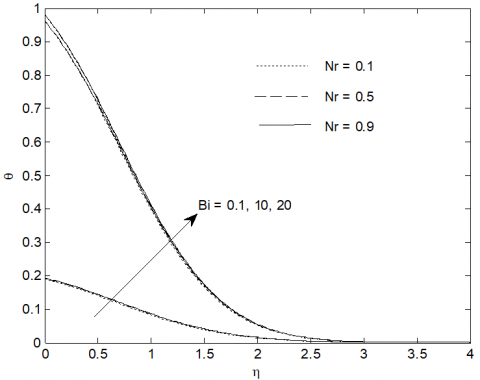Figure 2. Comparison of temperature profiles for Bi and Nr with RamReddy et al. 

Figure 3 exhibits the effect of magnetic parameter (M), porosity parameter (Kp) and chemical reaction parameter (Kc) on velocity distribution. The interaction of magnetic field with an electrically conducting flowing fluid induces a voltage across the magnetic field. The current generated by the induced voltage interacts with magnetic field and producing a resistive force to slow down the motion of the fluid. Therefore, an increase in M leads to decrease the velocity profiles in the flow domain. Further, porous medium resists the fluid flow also. It is also seen that the velocity increases with an increasing values of constructive chemical reaction parameter Kc (Kc<0) whereas reverse effect is observed is case of destructive chemical reaction parameter Kc (Kc>0).

## 3.png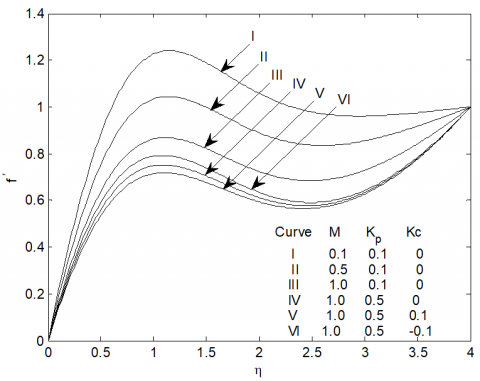Figure 3. Velocity profiles for various values of M, Kp and Kc with λ=Nr=Nc=Bi=Pr=ST=1.0, Nt=Nb=0.45, Sc=0.6, Le=5.0, Q=R=Ec=0.1

Figure 4 reveals the influence of nanoparticle buoyancy ratio (Nr) and regular buoyancy ratio (Nc) on velocity distribution. It is observed that thermal boundary layer decreases with an increasing value of Nr and increases with increasing values of Nc.

## 4.png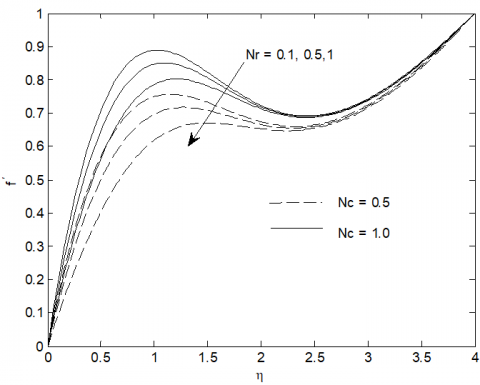Figure 4. Velocity profiles for various values of Nc and Nr with M=Kp=0.5, λ=Bi=Pr=ST=1.0, Nt=Nb=0.45, Sc=0.6, Le=5.0, Q=R=Ec=Kc=0.1

## 5.pngFigure 5. Velocity profiles for various values of Bi and λ with M=Kp=0.5, Nc=Nr=Pr=ST=1.0, Nt=Nb=0.45, Sc=0.6, Le=5.0, Q=R=Ec=Kc=0.1

## 6.pngFigure 6. Temperature profiles for various values of Nb and Nt with M=Kp=Q=R=Ec=Kc=0.1, λ=Bi=Pr=ST=Nr=Nc=1.0, Sc=0.6, Le=10.0

Figure 5 exhibits the impact of Biot number (Bi) and mixed convection parameter (λ) on velocity profiles. It is observed that both the parameters enhance the velocity. The Biot number is the ratio of momentum diffusivity through v and thermal diffusivity through k. Here, momentum diffusivity is accelerated overriding the thermal conductivity of nanofluid, resulting accelerated fluid motion.

From Figure 6 it is clear that Brownian motion (Nb) and thermophoresis parameter Nt increase the temperature indicating more dispersion of thermal energy into flow domain. It is also revealed that thermal energy is dissipated into flow due to concentration and temperature differences between boundary layers and corresponding ambient conditions as well as Brownian diffusion coefficient and thermophoresis rather than kinematic viscosity (υ) of the base fluid responsible for momentum diffusivity.

## 7.png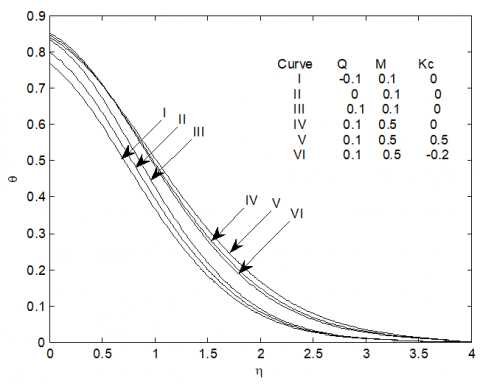Figure 7. Temperature profiles for various values of M, Q and Kc with Nb=Nt= 0.45, λ=Bi=Pr=ST=Nr=NC=1.0, Sc=0.6,Le=10.0, R=Ec=KP=0.1

## 8.png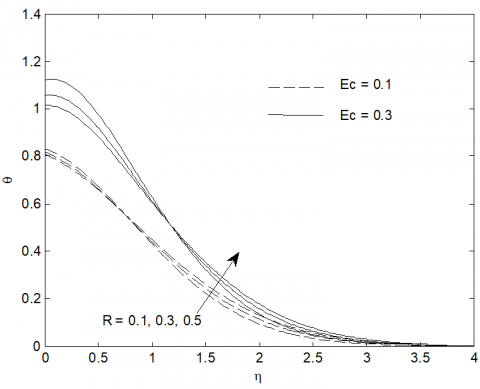Figure 8. Temperature profiles for various values of R and Ec with M=Kp=Q=KC=0.1,λ=Bi=Pr ST=Nr=Nc=1.0, Nb=Nt= 0.45, Sc=0.6, Le=10.0

Figure 7 depicts the influence of magnetic parameter (M), heat source/sink parameter (Q) and chemical reaction parameter (Kc) on temperature distributions. It is noticed that an increase in the value of magnetic parameter enhances the temperature of the fluid. The temperature profiles increase with heat source parameter Q(Q>0) on the contrary, the temperature decreases with heat sink parameter Q(Q<0). It is also seen that the temperature increases with an increase in the destructive chemical reaction parameter Kc(Kc>0) and decreases in case of constructive chemical reaction parameter Kc(Kc<0). It is natural in the process of exothermic reaction; the heat energy is evolved that enhances the fluid temperature. The reverse thermo-physical conditions is observed for endothermic reaction.

Figure 8 presents the effect of thermal radiation parameter (R) and Eckert number (Ec) on the temperature profiles. The effect of radiation parameter near the bounding surface (0<η<0.65) is to decrease the temperature and beyond, the reverse effect is observed.  The point of intersection of the profiles drifted away from the boundary with higher value of Ec.

## 9.png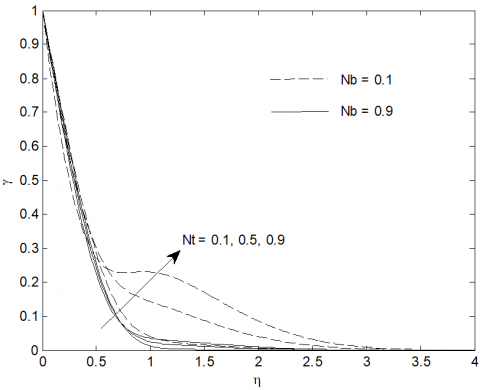Figure 9. Volume fraction profiles for various values of Nb and Nt with M=Kp=Q=KC=R=Ec=0.1, λ=Bi=Pr ST=Nr=Nc=1.0, Sc=0.6, Le=10.0

Figure 9 is plotted to explain the effects of Brownian motion (Nb) and thermophoresis parameter (Nt) on volume fraction profiles. A sharp fall in volume fraction is seen with the variation of Nb and Nt except some anomalies in case of slightly increased value of Nt equals to 0.5, 0.9 where, for which the fall slows down when 0.6<η<1.9. This indicates that increase in Brownian motion resulting higher energy exchange is prevented.

## 10.pngFigure 10. Volume fraction profiles for various values of Le and Kc with M=Kp=Q= R=Ec=0.1, Nb=Nt= 0.45, Bi=Pr ST=Nr=Nc=1.0, Sc=0.6

## 11.png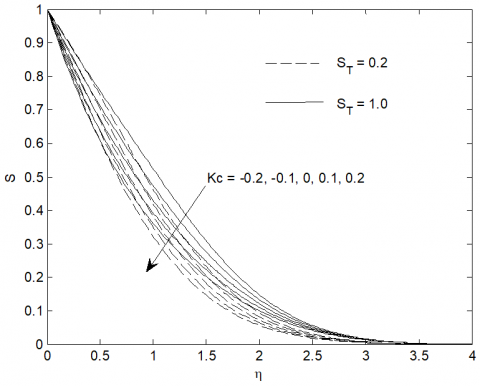Figure 11. Concentration profiles for various values of Kc and ST with M=Kp=Q=R=Ec=0.1, Nb=Nt= 0.45, λ=Bi=Pr Nr=Nc=1.0, Sc=0.6, Le=10.0

Figure 10 presents the effects of Lewis number (Le) and chemical reaction parameter (Kc) on volume fraction profiles. A sharp fall in volume fraction occurs due to higher value of Lewis number. One remarkable feature is that the volume fraction increases under the influence of exothermic reaction (Kc>0) and decreases for endothermic reaction (Kc<0).

Figure 11 depicts the effects of chemical reaction parameter (Kc) and Soret number (ST) on concentration profiles. It is observed that destructive chemical reaction parameter Kc (Kc>0) decreases the concentration profiles while concentration increases with increasing values of generative chemical reaction parameter Kc (Kc<0). It is also seen that an increase in the Soret number results in a significant increase in the concentration distributions within the solutal boundary layer.

Figure 12 displays the results of Schmidt number (Sc) and mixed convection parameter (λ) on concentration profiles.  The higher Sc representing heavier species lowers down the concentration level at all the layers when λ<1, whereas reverse effect is observed in case of λ>1.

## 12.pngFigure 12. Concentration profiles for various values of Sc and λ with M=Kp=Q=R=Ec=Kc=0.1, Nb=Nt= 0.45, Bi=Pr= Nr=Nc=1.0, Le=10

Table 2 exhibits the values of skin-friction coefficient f’’(0), heat transfer coefficient -θ’(0), regular Sherwood number -S’(0) and nanoparticle Sherwood number -γ’(0). It is found that all these surface criteria increase with increasing values of mixed convection parameter and Biot number whereas reverse effect is observed in case of magnetic parameter. Further, it is noticed that Nusselt number increases with increase in radiation parameter while skin-friction coefficient, regular Sherwood number and Sherwood number decrease with increase in thermal radiation parameter. On careful observation, it is seen that a significant reduction in heat transfer rate at the plate increases with Eckert number. On the other hand the skin friction coefficient, regular Sherwood number and Sherwood number increase with the increasing values of Eckert number. It is also noted that for destructive chemical reaction parameter Kc (Kc>0), the regular Sherwood number increases with an increase in Kc but skin friction coefficient, Nusselt number and nanoparticle Sherwood number increase with increase in constructive chemical reaction parameter Kc (Kc<0). One interesting result is that the rate of heat transfer at the wall decreases significantly with an increase in the heat source parameter Q (Q>0) whereas the reverse effect is observed in presence of heat sink parameter (Q>0). The temperature at the plate is θ’(0)=-Bi[1-θ(0)]. For Ec=0.1, θ(0)=0.8. Hence θ’(0)<0 when Bi>0. This indicates that due to higher value of Bi (Bi=5), the negative temperature at the plate could not be compensated due to the presence of heat source. Therefore, it is suggested that the value of Biot number to be reduced so that rate of heat transfer at the plate will increase.

Table 2. Variation of skin friction coefficients, heat transfer, nanoparticle mass transfer and regular mass transfer rates when Nr=Nc=Pr=ST=1.0, Kp=Nb=Nt=0.5, Sc=0.6, Le=10

 M R λ Bi Q Kc Ec f’’(0) -θ’(0) -S’(0) -γ’(0) 0.1 0.1 0.1 1 0.1 0.1 0.1 0.329590 0.128360 0.624443 0.962640 0.3 0.273681 0.111787 0.614570 0.904510 0.5 0.234912 0.098765 0.607985 0.859474 0.2 0.232762 0.118282 0.601947 0.849437 0.3 0.230929 0.137876 0.596791 0.840844 0.3 0.578370 0.178131 0.640187 1.091452 0.5 0.903096 0.198248 0.680273 1.260740 3 0.971418 0.223829 0.692468 1.297981 5 0.987150 0.229749 0.695277 1.306450 -0.1 0.935168 0.366736 0.635465 1.220796 0.2 1.017921 0.150520 0.729244 1.353885 0.3 1.052848 0.061865 0.766710 1.405225 -0.1 1.104324 0.159304 0.421675 1.397041 -0.2 1.166114 0.164769 0.219907 1.426968 0.2 0.986830 0.147087 0.852206 1.338019 0.3 0.961036 0.144160 0.961592 1.324746 0.2 1.007067 0.097796 0.872340 1.370280 0.3 1.028604 0.044253 0.894157 1.404843
5. Conclusion

From the present analysis the following conclusions are as follows:

The Lorentz force due to induced electromagnetic force reduces the thickness of momentum boundary layer whereas opposite effect is observed on the thermal boundary layer.

An increase in mixed convection parameter enhances the velocity profiles whereas it reduces the nanoparticle volume fraction as well as concentration profiles.

Heat transfer rate increases with increase in thermal radiation parameter, Biot number and mixed convection parameter.

Concentration profiles reduce with increasing Schmidt number for mixed convection parameter λ<1 but increase in case of λ>1.

A sharp fall in volume fraction is indicated for higher value of Lewis number.

The concentration decreases and volume fraction increases under the influence of exothermic reaction (Kc>0) while concentration increases and volume fraction decreases for endothermic reaction (Kc<0).

Nomenclature
 x, y co-ordinates along and normal to the plate u, v velocity components in x and y directions respectively J ratio between the effective heat capacity of the nanoparticle material and heat capacity of the fluid c constant k thermal conductivity Bi Biot number M magnetic parameter Pr Prandtl number Le Lewis number Ds solutal diffusivity DCT Soret diffusivity ST Soret number Ec Eckert number Q heat source/sink parameter R radiation parameter Kp porosity parameter Kc chemical reaction parameter $C_{\infty}$ ambient solutal concentration C solutal concentration $\phi_{w}$ nanoparticle volume fraction at the wall S dimensionless concentration $\phi$ nanoparticle volume fraction $\phi_{\infty}$ ambient nanoparticle volume fraction g acceleration due to gravity $S_{1}$ internal heat generation/absorption Nb Brownian motion parameter DB coefficient of Brownian diffusivity DT thermophoretic diffusion coefficient Hf convective heat transfer coefficient Sc Schmidt number Grx local Grashof number Nux local Nusselt number NC regular buoyancy ratio η similarity variables Nr nanoparticle buoyancy ratio Nt thermophoresis parameter λ mixed convection parameter μ dynamic viscosity v kinematic viscosity ψ steam function f dimensionless steam function T temperature θ dimensionless temperature Tf temperature of the hot fluid ρ density of the fluid T∞ ambient temperature ρf∞ density of the base fluid u∞ characteristics velocity αm thermal diffusivity of nanoparticle βT volumetric thermal expansion coefficient βc volumetric solutal expansion oefficient ρp mass density of the nanoparticle (ρc)f heat capacity of the fluid Rex local Reynolds number shx local Sherwood number local Sherwood number qn mass flux of nanoparticle at the wall qm regular mass flux at the wall qw heat flux at the wall τw shear stress at the wall (ρc)p effective heat capacity of the nanoparticle NShx local nanoparticle Sherwood number

Superscripts

‘differentiation with respect to $\eta$

References

 Choi, S. (1995). Enhancing thermal conductivity of fluids with nanoparticle. D.A. Siginer, H.P. Wang (Eds.), Developments and Applications of Non-Newtonian Flows. ASME MD Vol. 231 and FED, 66: 99-105.

 Choi, S., Zhang, Z.G., Yu, W., Lockwood, F.E., Grulke, E.A. (2001). Anomalously thermal conductivity enhancement in nanotube suspensions. Applied Physics Letters, 79(14): 2252-2254. https://doi.org/10.1063/1.1408272

 Buongiorno, J. (2006). Convective transport in nanofluids. Journal of Heat Transfer, 128(3): 240-250. https://doi.org/10.1115/1.2150834

 Navier, C.L.M.H. (1823). Memoir on the laws of the Movement of Fluids. Memoirs of the Royal Academy of Sciences of the Institute of France, 6: 389-440.

 Kuznetsov, A.V., Nield, D.A. (2010). Natural convective boundary-layer flow of a nanofluid past a vertical plate. International Journal of Thermal Sciences, 49(2): 243-247. https://doi.org/10.1016/j.ijthermalsci.2009.07.015

 Hussain, S.M., Sharma, R., Seth, G.S., Mishra, M.R. (2018). Thermal radiation impact on boundary layer dissipative flow of magneto-nanofluid over an exponentially stretching sheet. International Journal of Heat and Technology, 36(4): 1163-1173. https://doi.org10.18280/ijht.360402

 Nayak, M.K. (2016). Steady MHD flow and heat transfer on stretched vertical permeable surface in presence of heat generation/absorption, thermal radiation and chemical reaction. Modelling, Measurement and Control B, 85(1): 91-104.

 Swain, K., Parida, S.K., Dash, G.C. (2017). MHD heat and mass transfer on stretching sheet with variable fluid properties in porous medium. AMSE Journals, Modelling B, 86(3): 706-726.

 Pal, D., Chatterjee, S. (2013). Soret and Dufour effects on MHD convective heat and mass transfer of a power-law fluid over an inclined plate with variable thermal conductivity in a porous medium. Applied Mathematics and Computation, 219: 7556-7574. https://doi.org/10.1016/j.amc.2012.10.119

 Makinde, O.D., Aziz, A. (2011). Boundary layer flow of a nanofluid past a stretching sheet with a convective boundary condition. International Journal of Thermal Science, 50: 1326-1332. https://doi.org/10.1016/j.ijthermalsci.2011.02.019

 RamReddy, C., Murthy, P.V.S.N., Chamkha, A.J., Rashad, A.M. (2013). Soret effect on mixed convection flow in a nanofluid under convective boundary condition. International Journal of Heat and Mass Transfer, 64: 384-392. https://doi.org/10.1016/j.ijheatmasstransfer.2013.04.032

 Nayak, A., Panda, S., Phukan, D.K. (2014). Soret and Dufour effects on mixed convection unsteady MHD boundary layer flow over stretching sheet in porous medium with chemically reactive species. Applied Mathematics and Mechanics, 35(7): 849-862. https://doi.org/10.1007/s10483-014-1830-9

 Swain, K., Parida, S.K., Dash, G.C. (2018). MHD flow of viscoelastic nanofluid over a stretching sheet in a porous medium with heat source and chemical reaction. Annals of Chemistry - Materials Science, 42(1): 7-21. https://doi.org10.3166/acsm.42.7-21

 Bhargava, R., Goyal, M. (2014). MHD non-Newtonian nanofluid flow over a permeable stretching sheet with heat generation and velocity slip. International Journal of Mechanical and Mechatronics Engineering, 8(6): 910-916.

 Barik, R.N., Dash, G.C., Rath, P.K. (2015). Chemical reaction and heat source effects on MHD flow of visco-elastic fluid past an exponentially accelerated vertical plate embedded in a porous medium. AMSE Journals, Modelling B, 84(2): 80-104.

 Nayak, M.K., Dash, G.C., Singh, L.P. (2015). Flow and mass transfer analysis of a micropolar fluid in a vertical channel with heat source and chemical reaction. AMSE Journals, Modelling B, 84(1): 69-91.

 Kandasamy, R., Jeyabalan, C., Prabhu, K.S. (2016). Nanoparticle volume fraction with heat and mass transfer on MHD mixed convection flow in a nanofluid in the presence of thermo-diffusion under convective boundary condition. Applied Nanoscience, 6(2): 287-300. https://doi.org/10.1007/s13204-015-0435-5

 Das, S.K., Choi, S.U.S., Yu, W., Pradeep, T. (2007). Nano Fluids Science and Technology. Wiley, 261-262.

 Aziz, A. (2009). A similarity solution for laminar thermal boundary layer over a flat plate with a convective surface boundary condition. Communications in Nonlinear Science and Numerical Simulation, 14(4): 1064-1068. https://doi.org/10.1016/j.cnsns.2008.05.003